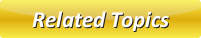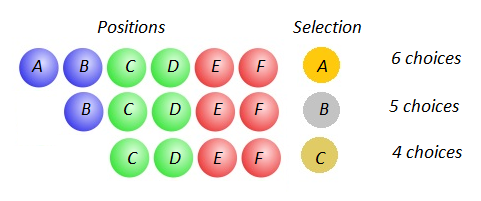# Permutation

In Mathematics, the permutation can be explained as the arrangement of objects in a particular order. It is an ordered Combination where order of arrangement is important. It is the all possible ways of doing something where order is taken into consideration.

Let us consider an example. For instance, there are 6 teams A, B, C, D, E and F participating in a contest. In how many ways can we award a 1st $(Gold)$, $2nd\ (Silver)$ and $3rd\ (Bronze)$ place prize among six teams?Permutations are used here as the order we give out the medals matters:

• Gold medal: 6 choices: A B C D E F. Example, A wins the contest for the Gold.
• Silver medal: 5 choices: B C D E F. Example, B wins the contest for the silver.
• Bronze medal: 4 choices: C D E F. Example, C wins the contest for the bronze.

First, we had 6 choices, then 5 and then 4. Total no. of options: $6 \times 5 \times 4$ = 120.

We should order three teams out of 6 teams. For doing this, we began with all 6 options and took them away one at a time $(5,\ then\ 4)$ until all medals are all given away.

We know that 6! = $6 \times 5 \times 4 \times 3 \times 2 \times 1$

And, 3! = 3 $\times 2 \times 1$

So, while doing $\frac{6!}{3!}$ we get:
$\frac{6!}{3!}$ = $\frac{(6 \times 5 \times 4 \times 3 \times 2 \times 1)}{(3 \times 2 \times 1)}$ = $6 \times 5 \times 4$ = 120

To derive a general formula for permutation, let us see how we can represent $\frac{6!}{3!}$ in total number of teams and number of medals.

$\frac{6!}{3!}$ can also be written as $\frac{6!}{(6-3)!}$.

The total number of teams contesting is 6, the total number of teams awarded are 3.

If n represents total number of teams, r represents number of teams awarded, and then the number of ways the teams can be ordered can be given by $\frac{n!}{(n-r)!}$. Here the same team cannot take two awards. So the order is not repeated.

When we consider examples like licence plate, password or codes, the objects $(terms\ or\ numbers)$ can repeat in the order. Such arrangements are considered as permutations with repetitions.

When we choose r of n things, then the permutations are $n \times n \times n...(r\ times)$ = nr

The notation for permutation is P(n, r) or nPr or nPr.

## Permutation Formula

Steps to find the permutation:

1. Identify if the arrangement is with repetition or without repetition.

2. If it is with repetition, then the permutation of selecting is calculated by:

$n \times n \times n...(r\ times)$ = nr

where, n is the total number of objects and r is the number of objects in arrangement.

3. If it is an arrangement without repetition, then the permutation of selecting is calculated by:
$P(n,\ r)$ or nPr or nPr = $\frac{n!}{(n-r)!}$

where, n is the total number of objects and r is the number of objects in arrangement.

## Permutation Solved Problems

Example 1: What is the total number of possible 3-letter arrangements of the letters r, i, g, h, t if each letter is used only once in each arrangement?

Solution: Since the arrangement has no repetitions, we find the permutation without repetitions.

Here, n = Total number of letters given = 5

r = Number of letters chosen = 3

Then the number of possible ways is given by:
P(n,r) = $\frac{n!}{(n-r)!}$

= $\frac{5!}{(5-3)!}$ = $\frac{5!}{2!}$ = $\frac{(5 \times 4 \times 3 \times 2 \times 1)}{(2 \times 1)}$ = 60

There are 60 ways possible 3 - letter arrangement from r, i, g, h, t if each letter is used only once.

Example 2: In how many ways can a 3 digit password can be set?

Solution: A password can be set with repetition of digits. So the arrangement is with repetition.

Here we have total number of digits $( 0,\ 1,\ 2...9)$, n = 10

and the number of digits chosen, r = 3.

Thus the possible ways is given by:
$P(n,\ r) = n \times n \times n...(r\ times)$ = nr

= 103 = 1000 ways.
Thus there are 1000 ways to set a 3 digit password.

Latest Articles

Average Acceleration Calculator

Average acceleration is the object's change in speed for a specific given time period. ...

Free Fall Calculator

When an object falls into the ground due to planet's own gravitational force is known a...

Permutation

In Mathematics, the permutation can be explained as the arrangement of objects in a particular order. It is an ordered...

Perimeter of Rectangle

A rectangle can be explained as a 4-sided quadrilateral which contains equal opposite sides. In a rectangle

Perimeter of Triangle

A three sided polygon which has three vertices and three angles is called a triangle. Equilateral triangle...